Latest Banking jobs   »   Reasoning Questions: Topics, Practice Set PDF & Reasoning Quiz For Bank Exams   »   Reasoning Ability Quiz For SBI Clerk...

# Reasoning Ability Quiz For SBI Clerk Prelims 2022- 4th May

Directions (1-5): Study the following data carefully and answer the questions accordingly.
In a certain code language:
‘College quarter class basic’ is coded as ‘dd bs nn cn’
‘Rank basic input adjust’ is coded as ‘us bs zj zx’
‘Rank entry class basic’ is coded as ‘us je nn bs’
‘College open entry class’ is coded as ‘nn cn tt je’

Q1. What is the code for ‘open’?
(a) nn
(b) je
(c) cn
(d) tt
(e) None of these

Q2. Which of the following is coded as ‘dd’?
(a) College
(b) Basic
(c) Quarter
(d) Class
(e) None of these

Q3.Which of the following is the possible code for ‘basic manners’?
(a) nn du
(b) gn bs
(c) vb gj
(d) cn nn
(e) None of these

Q4. What is the code for ‘open entry’?
(a) je cn
(b) tt bs
(c) nn us
(d) je tt
(e) None of these

Q5. Which of the following is coded as ‘zx’?
(a) Basic
(b) Input
(d) Rank
(e) Either B or C

Directions (6-10): Study the following information carefully and answer the given questions:
In a certain code language,
‘Level place slow’ is written as ‘ps jf hn’
‘Place catch slow’ is written as ‘st hn jf’
‘Bike place common’ is written as ‘jf pt to’
‘Slow catch place’ is written as ‘hn st jf’

Q6. Which of the following is the code for ‘catch’?
(a) Either ‘st’ or ‘jf’
(b) hn
(c) st
(d)jf
(e) None of these

Q7. If ‘Start bike’ is coded as ‘to mn’, then which of the following is the code for ‘common sense’?
(a) mn hn
(b) yt bn
(c) hu pt
(d) jf st
(e) None of these

Q8. Which of the following is coded as ‘ps’?
(a) Place
(b) Level
(c) Slow
(d) Catch
(e) None of these

Q9. What is the code for ‘catch slow level’?
(a) st hn to
(b) jf st pt
(c) st ps hn
(d) jf ps fg
(e) None of these

Q10. Four from the following are similar in a certain way and forms a group. Find out the one, which does not belong to that group.
(a) st
(b) ps
(c) hn
(d) jf
(e) pt

Directions (11-15): Study the following data carefully and answer the questions accordingly.
In a certain code language:
‘Set you the praise’ is written as ‘re fi zo na’,
‘Help to set you’ is written as ‘so re na di’,
‘Place cast to set the praise’ is written as ‘ge fi na di ke zo’,
‘Help to cast’ is written as ‘di so ge’.

Q11. Which among the following are coded as ‘re di so’?
(a) Praise you to
(b) To cast you
(c) Set you to
(e) Can’t be determined

Q12. What will be the code for ‘place set you’?
(a) so di na
(b) ke na di
(c) na di so
(d) ke re na
(e) Cannot be determined

Q13. What will be the code for ‘praise’?
(a) ge
(b) re
(c) zo
(d) fi
(e) Cannot be determined

Q14. Which among the following is coded ‘so’?
(a) Cast
(b) To
(c) Set
(d) Help
(e) None is correct

Q15. What will be to code for ‘place’?
(a) di
(b) zo
(c) na
(d) ke
(e) Cannot be determined

Solutions

Solution (1-5):

Sol.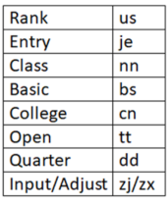S1. Ans. (d)

S2. Ans. (c)

S3. Ans. (b)

S4. Ans. (d)

S5. Ans. (e)

Solution (6-10):

Sol.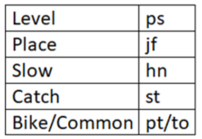S6. Ans. (c)

S7. Ans. (c)

S8. Ans. (b)

S9. Ans. (c)

S10. Ans. (e)

Solution (11-15):

Sol.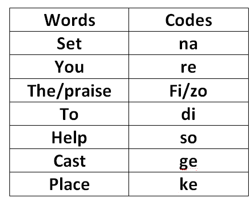S11. Ans. (d)

S12. Ans. (d)

S13. Ans. (e)

S14. Ans. (d)

S15. Ans. (d)#### Congratulations!

Incorrect details? Fill the form again here

•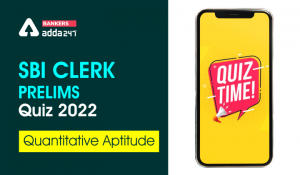Quantitative Aptitude Quiz For SBI Clerk...
•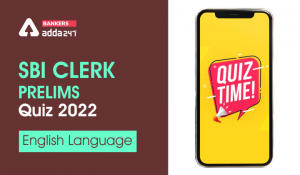English Quizzes For SBI Clerk Prelims 20...
•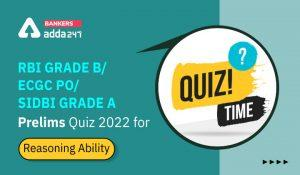Reasoning Ability Quiz For RBI Grade B/ ...
•Quantitative Aptitude Quiz For SBI Clerk...
•Quantitative Aptitude Quiz For SBI Clerk...
•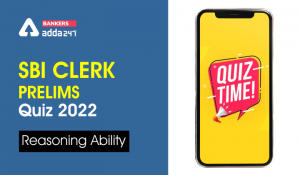Reasoning Ability Quiz For SBI Clerk Pre...